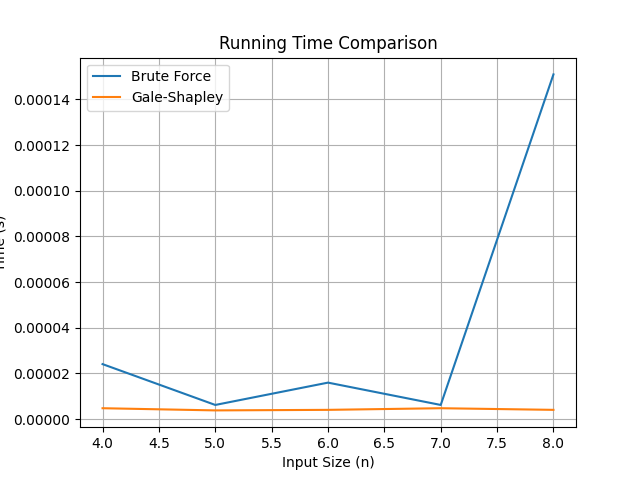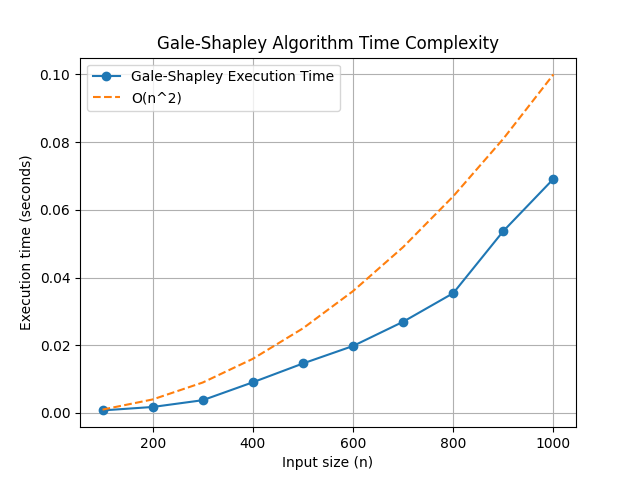# Complexity, Computation and Algorithms

Notes on Complexity theory, Theory of Computation and Algorithms

# Gale-Shapley Algorithm

## Input

• Two sets of agents: Men and Women
• Preference lists for each agent
• Men’s preferences: men_preferences
• Women’s preferences: women_preferences

## Output

• Stable matching: matching

## Initialize

• Initialize an empty matching matching
• Create an empty list men_proposals to keep track of men’s proposals

## While there are unengaged men

• Select an unengaged man m from Men
• Find the first woman w in m’s preference list whom m has not proposed to
• Mark m as having proposed to w
• If w is unengaged
• Engage m and w in a couple and add them to matching
• Else, if w is already engaged to m'
• If w prefers m to m'
• Break the engagement between m' and w
• Engage m and w in a couple and add them to matching
• Mark m' as unengaged
• Else, w rejects m

## End

• Return the stable matching matching

# Complexity Analysis

To prove that the Gale-Shapley algorithm has a time complexity of O(n^2), where ’n’ is the number of elements in each of the two sets being matched, we will analyze the worst-case scenario for both the proposal and acceptance phases.

In the proposal phase, each of the ’n’ men proposes to ’n’ women. This results in ’n’ proposals per man. Mathematically, we can represent this as:

[$\text{Number of proposals in proposal phase} = n \times n = n^2$]

In the acceptance phase, each of the ’n’ women considers proposals from ’n’ men. This results in ’n’ comparisons per woman. Mathematically, we can represent this as:

[$\text{Number of comparisons in acceptance phase} = n \times n = n^2$]

Since both the proposal and acceptance phases involve a total of ’n^2’ operations, we can add them together to find the total number of operations in the worst case:

[$\text{Total number of operations} = \text{Number of proposals in proposal phase} + \text{Number of comparisons in acceptance phase} = n^2 + n^2 = 2n^2$]

To express this in big O notation, we drop the constant factor (2) and write:

[$\text{Time complexity} = O(n^2)$]

Therefore, we have mathematically proven that the Gale-Shapley algorithm has a time complexity of O(n^2).

# Empirical Analysis

Running Gale-Shapely against a brute-force code, we have the following graph.We see that Gale-Shapely grows rises very little time wise as input size grows while brute-force grows quasi-exponentially (I do not mean mathematically exponential, but it is not linear either, looks closer to exponential).

The Brute-Force code I used has the following pseudo-code.

function bruteForceStableMarriage(Preferences):
Initialize an empty list for all possible permutations of marriages
Generate all possible permutations of marriages

for each permutation in allPermutations:
if isStable(permutation, Preferences):
return permutation

# If no stable marriage is found
return "No stable marriage exists"

function isStable(permutation, Preferences):
for each man in permutation:
for each woman in permutation:
if man prefers woman over his current partner in permutation:
if woman prefers man over her current partner in permutation:
return false
return true


Now looking at the code below where I have implemented Gale-Shapely, and iteratively increased the input size and charted it agaisnt time to run, we see that the time taken by the algorithm also charts a similar curve as [$n^2$], which is what we expect since we proved that previously.## Source code

(some errors might exist, I have not standardised the output format yet)

Source: https://theoryofcomputation.xyz/blog/gale-shapely/, 0001-01-01